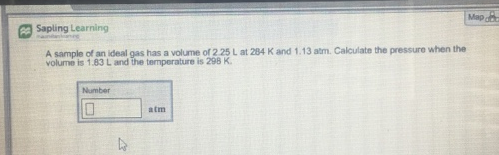Chemistry Practice Problems Chemistry Gas Laws Practice Problems Solution: A sample of an ideal gas has a volume or 2 25 L at...

# Solution: A sample of an ideal gas has a volume or 2 25 L at 284 K and 1.13 atm. Calculate the pressure when the volume is 1.83 L and the temperature is 298 K

###### Problem

A sample of an ideal gas has a volume or 2 25 L at 284 K and 1.13 atm. Calculate the pressure when the volume is 1.83 L and the temperature is 298 KView Complete Written Solution

Chemistry Gas Laws

Chemistry Gas Laws

#### Q. A sample of an ideal gas has a volume or 2.25 L at 284 K and 1.13 atm. Calculate the pressure when the volume is 1.83 L and the temperature is 298 K.

Solved • Fri Oct 05 2018 14:27:18 GMT-0400 (EDT)

Chemistry Gas Laws

#### Q. A sample of neon gas at 305 K and 0.372 atm occupies a volume of 1.89 L. If the pressure of the gas is increased, while at the same time it is heated ...

Solved • Wed Sep 12 2018 11:38:19 GMT-0400 (EDT)

Chemistry Gas Laws

#### Q. An ideal gas is allowed to expand from 9.00 L to 76.5 L at constant temperature. By what factor does the volume increase? The pressure will           ...

Solved • Wed Sep 12 2018 10:46:01 GMT-0400 (EDT)

Chemistry Gas Laws

#### Q. In this problem, your answers mathematical expressions involving a variable. a) Consider an ideal gas with an absolute temperature of T1. To what temp...

Solved • Tue Sep 11 2018 13:36:15 GMT-0400 (EDT)